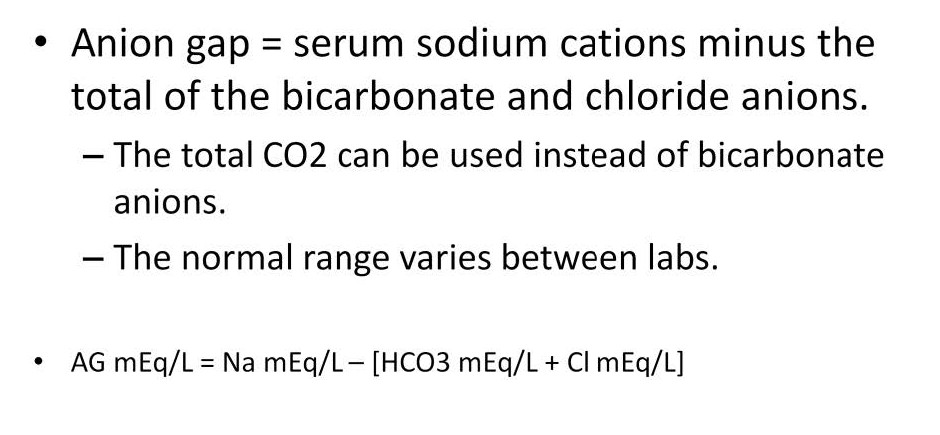### Anion Gap: Abbreviated FormulaAn abbreviated formula for the AG subtracts the total of the 2 major anions (Cl and HCO3) from the major cation sodium (Na). This is the formula used for the values described herein:
Na mEq/L – (Cl mEq/L + HCO3 mEq/L) = anion gap mEq/L
Example from blood serum: 135 – (100 + 24) = 11
The normal range varies depending on the type of testing equipment used at each laboratory. All of the AG values described herein were tested using blood serum and ion selective electrodes. However there is a wide range of analyzers that can result in a wide variation of anion gap measurements. For example, whole blood tests for the anion gap are not equivalent to serum measurements because of interference by formed elements in the blood. Also many laboratories include the potassium as part of the calculation and may also use the total carbon dioxide instead of the bicarbonate level. For example, test results from a whole blood sample that uses potassium and total CO2 in the formula may be different from the serum test and formula drawn on the same patient. This is a different formula:
(Na mEq/L + K mEq/L) – (Cl mEq/L + TCO2 mEq/L) = anion gap mEq/L
Example from whole blood: (135 + 5) – (100 + 25) = 15

#### CategoriesPerfusion Theory is an educational platform for the Oxygen Pressure Field Theory (OPFT). August Krogh’s theoretical concept of the oxygen pressure field is explained and then applied to clinical applications in perfusion practice.